Courses

# SSC CHSL Mock Test -3

## 100 Questions MCQ Test SSC CHSL Mock Test Series | SSC CHSL Mock Test -3

Description
This mock test of SSC CHSL Mock Test -3 for SSC helps you for every SSC entrance exam. This contains 100 Multiple Choice Questions for SSC SSC CHSL Mock Test -3 (mcq) to study with solutions a complete question bank. The solved questions answers in this SSC CHSL Mock Test -3 quiz give you a good mix of easy questions and tough questions. SSC students definitely take this SSC CHSL Mock Test -3 exercise for a better result in the exam. You can find other SSC CHSL Mock Test -3 extra questions, long questions & short questions for SSC on EduRev as well by searching above.
QUESTION: 1

### In the following question, some part of the sentence has errors and some have none. Find out which part of a sentence has an error and mark that part as your answer. If there is no error, then mark the answer as 'No error'. Q. Even after hours of / calling the salesman he / never showed up.

Solution:

There are no grammatical errors in the given sentence. Therefore, the correct answer is option 4.

QUESTION: 2

### In the following question, some part of the sentence has errors and some have none. Find out which part of a sentence has an error and mark that part as your answer. If there is no error, then mark the answer as 'No error'. Q. Hiten called me about / for an afternoon / trip to the lake.

Solution:

'About' is a preposition that means 'concerning something'. The phrase calling about is not a correct phrase. The correct form would be 'called around' or just 'called'. Therefore, the correct answer is option 1.

QUESTION: 3

### In the following sentence, a part of the sentence is underlined. Below are given alternatives to the underlined part, which may improve the sentence. Choose the correct alternative. In case no improvement is needed, choose the alternative that indicates No improvement needed. Q. All person in the world must pay attention to this fact.

Solution:

All is a determiner pronoun that is used to denote a group of people. Since the sentence uses the word 'person' after the word all, it cannot be correct. There must be a word that can be applicable to the singular person which is given by 'every', which represents each single quantity of something. Therefore, the correct answer is option 1.

QUESTION: 4

In the following sentence, a part of the sentence is underlined. Below are given alternatives to the underlined part, which may improve the sentence. Choose the correct alternative. In case no improvement is needed, choose the alternative that indicates No improvement needed.

Q. The statue was casted in pure gold and was studded with diamonds.

Solution:

The past tense form of the verb 'to cast' is cast and not casted. Therefore, the correct answer is option 1.
Improved sentence - The statue was cast in pure gold and was studded with diamonds.
The forms of cast is
cast  cast  cast
like Put Put Put

QUESTION: 5

In the following question, the sentence with blank is to be filled with appropriate word. Select the correct alternative out of the four and indicate it by selecting the appropriate option.

Q. Previously, she was one of the three Deputy Director-Generals supporting the Director-General in ________ all programmes.

Solution:

Option 3 is the correct answer because, 'overseeing' means 'supervising (a person or their work), especially in an official capacity'. The verb 'supporting' suggests that the next verb must also be in progressive form to form the parallelism.

Let us take an example to understand it better:

• My mother is helping my brother in completing his homework.

Option 1 is wrong because, first form of verb is given which doesn't form a balance with the verb 'supporting'.

Option 2 is wrong because, participle form is given.

Option 4 is wrong because, second form of verb is given.

QUESTION: 6

A sentence has been given in Active/Passive Voice. Out of the four alternatives suggested, select the one which best expresses the same sentence in Passive/Active Voice.

Q. My mother gave me the pen you saw earlier.

Solution:

The subject and object here are 'my mother' and 'The pen'. The adverb phrase 'you saw earlier' provides more information about 'the pen' and should be kept as it is. The subject and the object exchange places. The past tense verb gave would become was+ past participle form given. So, the correct option is option 2.

QUESTION: 7

A sentence has been given in Active/Passive Voice. Out of the four alternatives suggested, select the one which best expresses the same sentence in Passive/Active Voice.

Solution:

The sentence is present in passive voice. Here, the subject and object are 'The king' and the 'enemy', who would exchange places with each other. Since both the past participle and past tense form of the verb 'to overpower' is 'overpowered', it will be used in place of 'was+past participle of the verb'.

Therefore, the correct answer is option 3.

QUESTION: 8

In the following question, a sentence has been given in Direct/Indirect speech. Out of the four alternatives suggested, select the one which best expresses the same sentence in Indirect/Direct speech.

Q. The Judge asked the accused if he had been caught red-handed.

Solution:

Correct option- 1
Clearly, the sentence is an indirect speech, so it is changed into a direct speech.

Present Perfect Changes to Past Perfect
a) Direct: "I have been to Gujarat", he told me.
b)  Indirect: He told me that he had been to Gujarat.

Correct sentence- The Judge said to the accused, "Have you been caught red handed?"

QUESTION: 9

Yusuf said to Dipti, ''I am a better bowler than you.''

Solution:

The rule when converting direct speech to indirect is to pay attention to each word and change it accordingly. The verb 'said' becomes 'told' in reported speech.

The sentence contains the first person indicative simple tense verb 'am', which, in reported speech becomes, third person indicative, simple past tense 'was'. Not plural 'were', as the noun is singular here.

Therefore, the correct answer is option 4.

QUESTION: 10

In the following question, an idiomatic expression or a proverb is underline. Select the alternative which best describes its use in the sentence.

Q. He is so hard headed that no one wants him in their team.

Solution:

The idiom 'hard headed' means 'stubborn' or 'unwilling to change an opinion or idea.' Thus option 1 is the correct answer.

QUESTION: 11

In the following question, out of the four alternatives, select the alternative which is the best substitute of the phrase.

Q. One who compiles a dictionary

Solution:

The meanings of the words are:
Imposter ⇒ a person who pretends to be someone else in order to deceive others, especially for fraudulent gain

Funambulist ⇒ a tightrope walker
Lexicographer ⇒ One who compiles a dictionary
Fanatical ⇒ filled with excessive and single-minded zeal
Thus option 3 is the correct answer.

QUESTION: 12

In the following question, out of the four alternatives, select the alternative which is the best substitute of the phrase.

Q. To speak negatively about someone or something

Solution:

The meanings of the words are:

Insipid ⇒ lacking flavour; weak or tasteless.

Mitigate ⇒ to  improve a painful, unpleasant, or negative situation

Infer ⇒ to conclude from implicit evidence

Malign ⇒ to speak negatively about someone or something

Thus option 4 is the correct answer.

QUESTION: 13

In the following question, out of the four alternatives, select the word similar in meaning to the word given.

Alleged

Solution:

The correct option is option 3.

Alleged means 'said, without proof, to have taken place or to have a specified illegal or undesirable quality'.

Unfeigned- genuine; sincere.
Authentic- of undisputed origin and not a copy

Putative- generally considered or reputed to be.

QUESTION: 14

In the following question, out of the four alternatives, select the word similar in meaning to the word given.

Intrigue

Solution:

The correct option is option 2.

Intrigue means 'arouse the curiosity or interest of; fascinate'.

Parry- answer (a question or accusation) evasively.

Fascinate- attract the strong attention and interest of (someone).

Repel- drive or force (an attack or attacker) back or away.

QUESTION: 15

In the following question, out of the five alternatives, select the one which is opposite in meaning of the given word.

Concomitant

Solution:

The correct option is 4, i.e. unrelated

The word ‘concomitant’ means to ‘to be associated’. The antonym of ‘concomitant’ is thus ‘unrelated’ which means something which is not related.

Valetudinarian’ means to be obsessive about health.
Unwieldy’ means to be heavy.
Fritter’ means to squander.

QUESTION: 16

In the following question, out of the four alternatives, select the word opposite in meaning to the word given.

Demur

Solution:

The correct option is option 3. Demur means 'raise objections or show reluctance'.

Dissent- the holding or expression of opinions at variance with those commonly or officially held.

Altercation- a noisy argument or disagreement, especially in public.

Concord- agreement or harmony between people or groups.

QUESTION: 17

​The sentence(s) given with blanks are to be filled with an appropriate word(s). Four alternatives are suggested for each question. For each question, choose the correct alternative and click the button corresponding to it.

Q. China demands the _________  of a part of Indian territory.

Solution:

Let's find out the meanings of the words:

Cult-a system of religious veneration devoted to a particular figure

Cession-giving up

Polity-a form or process of civil government

Ramification-an unwelcome consequence of an action

As we see from the above descriptions, only option 2 fits appropriately in the sentence.

Complete sentence:

China demands the cession of a part of Indian territory.

QUESTION: 18

The sentence(s) given with blanks are to be filled with an appropriate word(s). Four alternatives are suggested for each question. For each question, choose the correct alternative and click the button corresponding to it.

Q. The _________  person left behind him a widow with three children.

Solution:

Let us find the meanings of the following words:

Demanded-ask authoritatively or insisted on something

Controlled-to have control over something

Confused-unable to think clearly

Deceased-to have died

As seen from the description of the words given, a 'widow' means 'the wife of a dead man'. According to that, only option 4 seems appropriate.

Complete sentence:

The deceased person left behind him a widow with three children.

QUESTION: 19

In the following question, the first and the last parts of the sentences have been numbered 1 and 6. The rest of the sentence is split into four parts and named P, Q, R and S. These four parts are not given in their proper order. Read the following sentence and find out which of the four combinations is correct. Then mark the correct answer from the options given below.

1. The pictures were great and we
P. one definitely needs a successful
Q. a camera determines the perfection of
R. pictures and to make a good portfolio
S. were convinced that the quality of
6. photographer for oneself.

Solution:

The first statement must be S as it states what happened with 'we'. Q follows S as it answers the question 'quality of what'. R must follow Q to give a meaning. P must follow R as it states what needs to be done to make a good portfolio. Thus option 3 is the correct answer.

QUESTION: 20

Read the passage given below and then answer the questions given below the passage. Some words may be highlighted for your attention. Read carefully.

In the fast paced lives, we are becoming increasingly distanced. This is happening despite the fact that the population of the country is only rising and are one of the most populous places in the world. Hundreds of people pass each other in the roads, public places, work places and yet, we detachment seems to be growing. This does not account that there is no attachment, only that there is less attachment and more individualism. Coming from a collectivistic culture, where people lived and thrived as a group, we are now moving toward an individualistic culture.

Q. What life seems to be the focus in the passage?

Solution:

The passage mentions “fast-paced life” which is most appropriately observed in cities or more broadly, in the urban areas. The answer is thus, B- urban life. Rural life, isolated life and coastal life are the other options and not correct since they are do not have fast paced life as a characteristic feature.

QUESTION: 21

Read the passage given below and then answer the questions given below the passage. Some words may be highlighted for your attention. Read carefully.

In the fast paced lives, we are becoming increasingly distanced. This is happening despite the fact that the population of the country is only rising and are one of the most populous places in the world. Hundreds of people pass each other in the roads, public places, work places and yet, we detachment seems to be growing. This does not account that there is no attachment, only that there is less attachment and more individualism. Coming from a collectivistic culture, where people lived and thrived as a group, we are now moving toward an individualistic culture.

Q. What dichotomy is being spoken about in the passage?

Solution:

The passage discusses the increasing growth of individualism in the cultures that were traditionally collectivistic and this indicates the dichotomy of the collectivistic and individualistic society comes forth. Hence, the answer is C- collectivistic and individualistic society. A- is not the answer since the urban life is pointed out but the difference of urban and rural societies is not the theme. B- is not the answer as there is no mention of the generational differences. D- is not the answer because there are no demarcations related to development mentioned in the passage.

QUESTION: 22

Read the passage given below and then answer the questions given below the passage. Some words may be highlighted for your attention. Read carefully.

In the fast paced lives, we are becoming increasingly distanced. This is happening despite the fact that the population of the country is only rising and are one of the most populous places in the world. Hundreds of people pass each other in the roads, public places, work places and yet, we detachment seems to be growing. This does not account that there is no attachment, only that there is less attachment and more individualism. Coming from a collectivistic culture, where people lived and thrived as a group, we are now moving toward an individualistic culture.

Q. What feeling is seen as primary in the individualistic society as mentioned in the passage?

Solution:

“Distanced” and “detachment” are the two feelings stated clearly in the passage. The answer is A- detachment. The other feelings also may be seen in the individualistic society but is not the answer because the passage does not mentions them.

QUESTION: 23

Read the passage given below and then answer the questions given below the passage. Some words may be highlighted for your attention. Read carefully.

In the fast paced lives, we are becoming increasingly distanced. This is happening despite the fact that the population of the country is only rising and are one of the most populous places in the world. Hundreds of people pass each other in the roads, public places, work places and yet, we detachment seems to be growing. This does not account that there is no attachment, only that there is less attachment and more individualism. Coming from a collectivistic culture, where people lived and thrived as a group, we are now moving toward an individualistic culture.

Q. What is the suggested direction of the society mentioned in the passage?

Solution:

The answer here is C- toward individualism, as the passage mentions the way due to changed life circumstances and scenarios the traditionally collectivistic societies are moving in the direction of individualism, away from their roots. A and B- are not the answer because there is no mention of any doom or destruction. D- is not the answer because technology is not a topic in the given passage.

QUESTION: 24

Read the passage given below and then answer the questions given below the passage. Some words may be highlighted for your attention. Read carefully.

In the fast paced lives, we are becoming increasingly distanced. This is happening despite the fact that the population of the country is only rising and are one of the most populous places in the world. Hundreds of people pass each other in the roads, public places, work places and yet, we detachment seems to be growing. This does not account that there is no attachment, only that there is less attachment and more individualism. Coming from a collectivistic culture, where people lived and thrived as a group, we are now moving toward an individualistic culture.

Q. What is the contradiction pointed out in the passage?

Solution:

The answer from the given options is clearly D- rising population and rising individualism, as the passage points at the booming population phenomena and instead of coming together, we are further drifting apart, even in societies that have been collectivistic in the past.

QUESTION: 25

In the following question, four words are given out of which one word is incorrectly spelt. Select the incorrectly spelt word.

Solution:

The correct option is option 1.

It is incorrectly spelt. The correct spelling is 'Deterrence'.

Its meaning is, 'the action of discouraging an action or event through instilling doubt or fear of the consequences'.

QUESTION: 26

In the following question, from the given alternative words, select the word which CANNOT be formed using the letters of the given word.

LINGUISTICS

Solution:

1) SUITINGS → LINGUISTICS, hence word can be formed.

2) UTILISING → LINGUISTICS, hence word can be formed.

3) LISTING → LINGUISTICS, hence word can be formed.

4) STICKS → LINGUISTICS, hence word cannot be formed (as letter K is not present).

Hence, STICKS is the correct alternative.

QUESTION: 27

In the following question, select the odd letters from the given alternatives.

Solution:

Here, in all groups except LFQ, when we consider numerical value of each letter, second letter is half the numerical value of first letter and third letter is thrice the numerical value of second letter.
1) PHX → P ÷ 2 = H, H × 3 = X
2) RIA → R ÷ 2 = I, I × 3 = A (26 + 1)
3) FCI → F ÷ 2 = C, C × 3 = I
4) LFQ → L ÷ 2 = F, F × 3 = R ≠ Q
Hence, ‘LFQ’ is the odd one.

QUESTION: 28

A series is given with one term missing. Select the correct alternative from the given ones that will complete the series.

31.2, 33.5, 28.9, 35.8, 26.6, ?

Solution: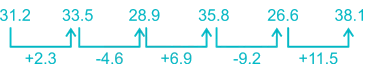Hence, ‘38.1’ will complete the given series.

QUESTION: 29

A man started walking from point A and walked 6 km towards the west and stopped at point B. From there, he turned left and walked 8 km to reach point C. Now, from point C, he moves 13 km to his opposite direction to reach point D. What is the shortest distance between point A and D?

Solution:

The diagram for the given direction problem is as follows: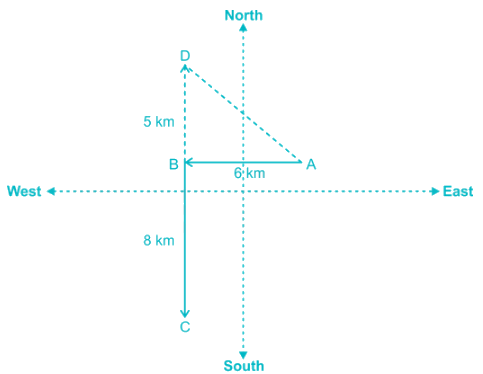(AD)2 = 25 + 36 = 61
Hence, the shortest distance between point A and D is √61 km.

QUESTION: 30

Arrange the given words in the sequence in which they occur in the dictionary.
1. Architectures
2. Aqua
3. Army
4. Architectonic

Solution:

The sequence in which they occur in the dictionary is as follows:
2. Aqua
4. Architectonic
1. Architectures
3. Army
Hence, ‘2413’ is the correct sequence.

QUESTION: 31

If 3 # 6 = 6, 5 # 9 = 8, then find the value of 6 # 8?

Solution:

Here, difference of numbers is taken and then twice of it is done.
3 # 6 = 6 → 6 – 3 = 3, 2 × 3 = 6
5 # 9 = 8 → 9 – 5 = 4, 2 × 4 = 8
6 # 8 = ? → 8 – 6 = 2, 2 × 2 = 4
Hence, 4 is the correct alternative.

QUESTION: 32

Correct the following equation by interchanging the two signs.

1286 ÷ 4 × 61 × 2 ÷ 2 + 63 – 121.5 = 324

Solution:

Given equation: 1286 ÷ 4 × 61 × 2 ÷ 2 + 63 – 121.5 = 324

Let’s check each option,

1) Interchanging + and – :
Equation will become 1286 ÷ 4 × 61 × 2 ÷ 2 - 63 + 121.5
= 321.5 × 61 × 1 – 63 + 121.5
= 19611.5 – 63 + 121.5
= 19548.5 + 121.5
= 19670

2) Interchanging × and + :
Equation will become 1286 ÷ 4 + 61 + 2 ÷ 2 × 63 – 121.5
= 321.5 + 61 + 1 × 63 – 121.5
= 321.5 + 61 + 63 – 121.5
= 324

3) Interchanging – and ÷ :
Equation will become 1286 - 4 × 61 × 2 - 2 + 63 ÷ 121.5
= 1286 – 4 × 61 × 2 – 2 + 63 ÷ 121.5
= 1286 – 488 – 2 + 0.52
= 796.52

4) Interchanging + and ÷ :
Equation will become 1286 + 4 × 61 × 2 + 2 ÷ 63 – 121.5
= 1286 + 4 × 61 × 2 + 0.032 – 121.5
= 1286 + 488 + 0.032 – 121.5
= 1652.532
Thus, using option 2, we get correct answer.

QUESTION: 33

A word is represented by only set of numbers as given in any one of the alternatives. The sets of numbers given in the alternatives are represented by two classes of alphabets as shown in the given two matrices. The columns and rows of Matrix-I are numbered from 0 to 4 and that of Matrix-II are numbered from 5 to 9. A letter from these matrices can be represented first by its row and next by its column, for example ‘E’ can be represented by 30, 34 etc. and ‘P’ can be represented by 55, 57, 95 etc. Similarly, you have to identify the set for the word ‘INDIAN’.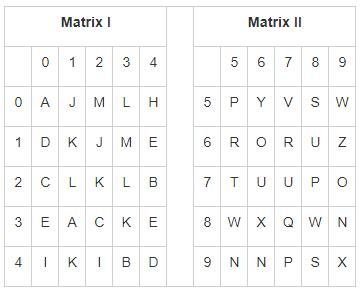Solution:

1) 42, 96, 44, 40, 00, 89 → I, N, D, I, A, N
2) 40, 96, 44, 40, 31, 13 → I, N, D, I, A, M
3) 78, 13, 10, 40, 00, 96 → P, M, D, I, A, N
4) 42, 96, 65, 40, 00, 89 → I, N, R, I, A, N
Hence, ‘42, 96, 44, 40, 00, 89’ represents the set for the word ‘INDIAN’.

QUESTION: 34

In the following question, select the related number from the given alternatives.

56 : 03 : 78 : ?

Solution:

The pattern followed here is:
56 : 03 → 5 × 6 = 30, Reverse of 30 = 03
Same pattern will be followed for ‘78 : ?’.
78 : ? → 7 × 8 = 56, Reverse Of 56 = 65

QUESTION: 35

In the following question, select the related letters/numbers from the given alternatives.

SWIFT : 59 ∷ DRUMS : ?

Solution:

Consider the English alphabetical series for the numerical value of letters:
The pattern followed here is:
S + W – I + F + T = 19 + 23 – 9 + 6 + 20 = 59
Same pattern will be followed for DRUMS : ?
D + R – U + M + S = 4 + 18 – 21 + 13 + 19 = 33
Hence, 33 is the correct alternative.

QUESTION: 36

If we form a cube by folding the given sheet of paper, how many persons will be on the face opposite to the face with three persons?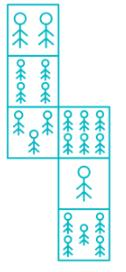Solution:

In the given figure, given faces should be opposite to each other:
2 persons should be opposite to 3 persons.
4 persons should be opposite to 1 persons.
6 persons should be opposite to 5 persons.
Hence, the answer is 2 persons.

QUESTION: 37

Study the following information carefully and answer the question based on it.

In the following figure, circle represents Whats-App, triangle represents Facebook and rectangle represents Google.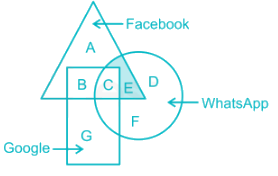Q. Which set of letters represents the combination of Whats-App and Facebook but not Google?

Solution:

Here, E represents the combination of Whats-App and Facebook but not Google.Hence, the answer is option 4.

QUESTION: 38

In a certain code language, TESTED is written as VBWOKW. How is WEDNES written in that code language?

Solution:

The pattern followed here is: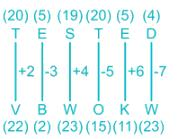Same pattern will be followed for WEDNES.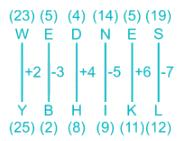Hence, WEDNES will be coded as YBHIKL.

QUESTION: 39

In the following question, select the odd word from the given alternatives.

Solution:

Here, all except Climatology are types of rainfall.
Hence, ‘Climatology’ is the odd one.

QUESTION: 40

Which one set of letters when sequentially placed at the gaps in the given letter series shall complete it?

p _ _ l p s _ _ _ s _ _

Solution:

By checking each option:
1) smmlppl → psml / psml / pspl
2) smplpml → psml / pspl / psml
3) pmmlpml → ppml / psml / psml
4) smmlpml → psml / psml / psml

QUESTION: 41

In the following question, select the odd number pair from the given alternatives.

Solution:

Here, difference of first and third digit is taken and then its square is taken to give second number.
1) 21, 25, 16 → 21 – 16 = 5, (5)2 = 25
2) 13, 12, 14 → 13 – 14 = -1, (-1)2 = 1 ≠ 12
3) 17, 121, 28 → 17 – 28 = -11, (-11)2 = 121
4) 11, 36, 17 → 11 – 17 = -6, (-6)2 = 36
Hence, ‘13, 12, 14’ is the odd one.

QUESTION: 42

Statements:
All papers are exams. Some exams are pages. No page is clean. Some cleans are ruled. Some ruled are not books.

Which of the following conclusion is definitely false?
I. Some pages are papers.
II. No clean is book.
III. All ruled can be books.
IV. Some exams are papers.

Solution:

The least possible Venn diagram for the given statements is as follows,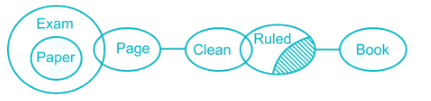I. Some pages are paper → it can be true.
II. No clean is book → it can be true.
III. All ruled can be books → it is definitely false.
IV. Some exams are paper → True (as shown in figure)
Hence, conclusion III is definitely false.

QUESTION: 43

In the Following Question, Select the related word from the given alternatives.

Q. Volley Ball : USA ∷ ? : England

Solution:

Volley ball is originated in USA. In the same way, Soccer is originated in England.
Hence, ‘Soccer’ is the correct alternative.

QUESTION: 44

In the following question, select the odd number pair from the given alternatives.

Solution:

Here, sum of digits of first and third number is taken and then their multiplication gives the middle number.

1) 34, 77, 56 → 3 + 4 = 7, 5 + 6 = 11, 7 × 11 = 77
2) 63, 45, 41 → 6 + 3 = 9, 4 + 1 = 5, 9 × 5 = 45
3) 81, 91, 28 → 8 + 1 = 9, 2 + 8 = 10, 9 × 10 = 90 ≠ 91
4) 28, 50, 32 → 2 + 8 = 10, 3 + 2 = 5, 10 × 5 = 50
Hence, ‘81, 91, 28’ is the odd one.

QUESTION: 45

Which one set of letters when sequentially placed at the gaps in the given letter series shall complete it?

l _ p _ _ q _ s l q _ _

Solution:

By checking each option:
1) qslqqs → llps / lqqs / lqqs
2) qslpps → lqps / lqps / lqps
3) qsqpps → lspq / pqps / lqps
4) qslppl → lspq / lspq / lqpl
Hence, ‘qslpps’ will complete the following series.

QUESTION: 46

If a mirror is placed on the line AB, then which of the answer figures is the right image of the given figure?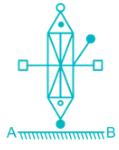Solution: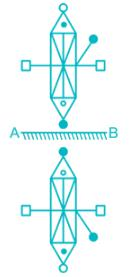Hence, the answer is option 1.

QUESTION: 47

Which one set of letters when sequentially placed at the gaps in the given letter series shall complete it?

a k _ _ a _ _ h a _ j _

Solution:

By checking each option:
1) jhkjkh → akjh / akjh / akjh
2) jhkjka → akjh / akjh / akja
3) jhkhkh → akjh / akhh / akjh
4) akkjkh → akak / akjh / akjh
Hence, ‘jhkjkh’ will complete the given series.

QUESTION: 48

Correct the following equation by changing multiply with any other sign.

8 + 63 – 48 ÷ 8 × 2 + 6 = 74

Solution:

Given equation: 8 + 63 – 48 ÷ 8 × 2 + 6 = 74

Let’s check each option,

1) Changing × with ÷ :
Equation will become: 8 + 63 – 48 ÷ 8 ÷ 2 + 6
= 8 + 63 – 48 ÷ 8 ÷ 2 + 6
= 8 + 63 - 3 + 6
= 74

2) Changing × with - :
Equation will become: 8 + 63 – 48 ÷ 8 - 2 + 6
= 8 + 63 - 6 - 2 + 6
= 71 - 2
= 69

3) Changing × with + :
Equation will become: 8 + 63 – 48 ÷ 8 + 2 + 6
= 8 + 63 - 6 + 2 + 6
= 71 + 2
= 73
Thus, using option 1, we get correct answer.

QUESTION: 49

Six utensils in a kitchen are tagged – R, M, N, P, T and W placed in ascending order (as per the size) from left to right. W is placed immediately left of P and immediately right of M. R is between N and T. T is somewhere to the left of M. N is the smallest utensil.

Which utensil is placed at the forth position (in ascending order)?

Solution:

Order and ranking is as follows:
N > R > T > M > W > P
Thus, M is at the forth position.

QUESTION: 50

A series is given with one term missing. Select the correct alternative from the given ones that will complete the series.

17, 24, 44, 78, ?

Solution: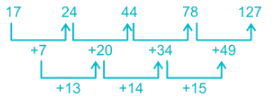QUESTION: 51

The shadow of building becomes 40 meter longer when the latitude of sun changes to 45° to 30° the height of building is?

Solution: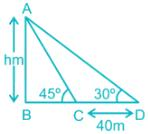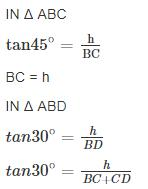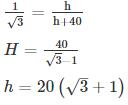QUESTION: 52

Simplify the expression {[√(x-3/5)]-5/3}5.

Solution:

Given that,
{[√(x-3/5)]-5/3}5
⇒ (x-3/5)1/2 × (-5/3) × 5
⇒ (x-3/5)-25/6
⇒ (x)-25/6 ×-3/5 = x5/2
∴ The required answer is x5/2

QUESTION: 53

Find the sum of all two digits numbers divisible by 4.

Solution:

Required numbers are: 12, 16, 20, ……, 96

This is an arithmetic progression series in which first term, a = 12 difference, d = 4 and last term l = 96. Let no. of terms in this series is n.
⇒ l = a + (n – 1) × d
⇒ 96 = 12 + (n – 1) × 4
⇒ (n – 1) × 4 = 84
⇒ n – 1 = 21
⇒ n = 22
∴ required sum = (n/2) × (a + l) = (22/2) × (12 + 96) = 11 × 108 = 1188

QUESTION: 54

The arithmetic mean of 9 numbers is 100 and the average of three of them is 40. What is the average of other six numbers?

Solution: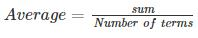⇒ Sum = Average × Number of terms
Let the sum of other six numbers be x, then as per question
Sum of 9 numbers = Sum of 3 numbers + sum of other 6 numbers
⇒ 100 × 9 = 40 × 3 + x
⇒ x = 900 – 120 = 780
∴ Average of other six numbers = 780/6 = 130

QUESTION: 55

Factorise - (x8 + x4y4 + y8)

Solution:

(x8 + x4y4 + y8)
x8 + 2 x4 y4 + y8 - x4 y4
(x4 + y4)2 - ( x2 y2)2
(x4 + y4 + x2 y2)( x4 + y4 - x2 y2)
[(x2 + y2)2 - (xy)2] ( x4 + y4 - x2 y2)
(x2 + x y + y2)( x2 - x y + y2) )( x4 + y4 - x2 y2)

QUESTION: 56

If X is 60% more than Y, then Y is what percentage less than X?

Solution:

Given,
⇒ X = Y + 60% of Y
⇒ X = Y + 0.6Y
⇒ X = 1.6Y
⇒ Y = (1/1.6)X
⇒ Y = 0.625X
⇒ Y = 62.5% of X
⇒ Y = X - (100 - 62.5)% of X
⇒ Y = X - 37.5% of X
∴ Y is 37.5% less than X

QUESTION: 57

The sum of the ages of 5 children born at the intervals of 2 years each is 60 years. What is the age of the youngest child?

Solution:

Let the age of children’ s be x, x + 2, x + 4, x + 6, x + 8
⇒ Sum of age = 60
⇒ x + x + 2 + x + 4 + x + 6 + x + 8 = 60
⇒ 5x + 20 = 60
⇒ 5x = 40
⇒ x = 8
∴ Age of youngest child = x = 8 years

QUESTION: 58

A shopkeeper bought a calculator for Rs. 400 and marked its price 37.5% above the original price. He announced a discount of 10% on the calculator. A boy bought the calculator at an additional discount of Rs. 15. How much profit did the shopkeeper earn on the calculator?

Solution:

Cost price of calculator = Rs. 400
Marked price = 400 + 37.5% of 400 = 400 + 3/8 × 400 = 400 + 150 = Rs. 550

Discount of 10% = 10% of 550 = 1/10 × 550 = Rs. 55
⇒ Selling price = 550 - 55 - 15 = Rs. 480
∴ Profit earned by shopkeeper = 480 - 400 = Rs. 80

QUESTION: 59

The difference between compound interest and simple interest on a certain amount of money at 5% per annum for 2 years is Rs. 45. Find the sum:

Solution:

Let the sum amount be ‘x’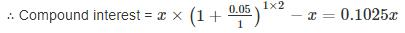Simple interest = (x × 2 × 5)/100 = 0.1x
According to the given conditions,
⇒ 0.1025x - 0.1x = 45
∴ x = 18,000
∴ The required sum is Rs. 18,000

QUESTION: 60

Vishnu travelled equal distance at speeds of 10 km/hr, 30 km/hr, and 8 km/hr, and took a total of 15.5 minutes to complete. Find the total distance he travelled, in km.

Solution:

Let he travelled x km at each speed.Time taken to cover x km at 10 kmph = x/10 hr

Time taken to cover x km at 30 kmph = x/30 hr

Time taken to cover x km at 8 kmph = x/8 hr

Total time taken = 15.5 minutes = 31/120 hr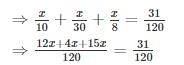⇒ 31x = 31
∴ x = 1
∴ Total distance covered in km = 1 + 1 + 1 = 3 km

QUESTION: 61

If 6 men working 8 hours a day earn Rs. 1680 per week, then 9 men working 6 hours a day will earn per week:

Solution:

Total hours of work done in a week = 6 × 8 × 7 = 336 hrs

Money earned by working 1 hr = 1680/336 = Rs. 5

Total money earned by 9 men working 6 hours a day = 5 × (9 × 6 × 7) = Rs. 1890

QUESTION: 62

Find the volume of a hexagonal prism if the surface area of the prism is 300√3 cmand base side is 5√3 cm.

Solution:

Let the height and base of the hexagonal prism are h and a respectively.

Surface area = 6ah + 3√3a2
⇒ 6 × 5√3h + 3√3(5√3)2
⇒ 30√3h + 225√3

Given Surface area = 300√3 through which we can find ‘h’
⇒ 30√3h + 225√3 = 300√3
⇒ √3(30h + 225) = 300√3
⇒ h = 75/30 = 5/2
∴ Volume of the prism = 3√3a2h/2
⇒ 3√3/2 × (5√3)2 × 5/2 = 281.25√3 cm3

QUESTION: 63

Find the equation of the line through the points (1, 2) and (5, 3).

Solution:

We know that,
Two point form of a line through the points (x1, y1) and (x2, y2) is (y - y1) = [(y2 - y1)/(x2 - x1)] × (x - x1)

The equation of the line through the points (1, 2) and (5, 3) is
⇒ (y - 2) = [(3 - 2)/(5 - 1)] × (x - 1)
⇒ (y - 2) = (1/4) × (x - 1)
⇒ 4y - 8 = x - 1
⇒ x - 4y + 7 = 0

QUESTION: 64

Find the fourth proportional to 28, 32 and 49.

Solution:

As we know that, Fourth proportional = (b × c)/a

Where a,b and c are the 1st, 2nd and 3rd elements of proportion respectively a = 28, b = 32 and c = 49
(32 × 49)/28 = 56

QUESTION: 65

A, B and C entered into a partnership and their share are in the ratio of 1/2 : 1/3 : 1/4. After 2 months, A withdraw half of his capital and after another 10 months a profit of Rs. 378 is divided among them. What is B’s share after 12 months?

Solution:

Ratio of initial investment of A, B and C = 1/2 : 1/3 : 1/4 = 6 : 4 : 3
Let A’s initial investment is = 6x
Let B’s initial investment is = 4x
Let C’s initial investment is = 3x
∴ A : B : C = (6x × 2 + 3x × 10) : (4x × 12) : (3x × 12)
⇒ (42x) : (48x) : (36x)
⇒ 42 : 48 : 36
⇒ 7 : 8 : 6
∴ B’s share in the profit = 378 × (8/21)
⇒ Rs. 144

QUESTION: 66

An angle is smaller than its supplementary angle by 50°. What is the measure of the angle?

Solution:

Let the measure of the angle be x°.
So, the supplementary angle = 180° - x°.
An angle is smaller than its supplementary angle by 50°.
So, we can write now,
(180° - x°) - x° = 50°
⇒ 2x° = 130°
⇒ x° = 65°.
∴ The required measure of the angle = 65°.

QUESTION: 67

How many gallons of each of a 4% and an 11% acid solutions should be mixed to obtain 35 gallons of a 7% solution?

Solution:

Let us denote by y gallons the amount of 4% solution.
⇒ The amount of solution of 11% acid solution = 35 - y gallons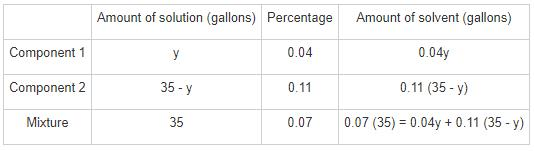Solving 0.07 (35) = 0.04y + 0.11 (35 - y),we get
y = 20 gallons
If y = 20 gallons; then the other amount, denoted by 35 - y must be 35 - 20 = 15 gallons.

QUESTION: 68

The unit’s digit is nine times the ten’s digit in a two-digit number. If 72 is added to the number, the digits interchange their places. Find the original number?

Solution:

Let the digit in unit’s place be x and digit in ten’s place be y
⇒ x = 9y and number = 10y + x
The number obtained by reversing the digits = 10x + y
⇒ 10y + x + 72 = 10x + y
⇒ 9y – 9x = – 72
⇒ y – x = – 8

Substituting x = 9y in the above equation, we get,
–8y = –8
⇒ y = 1
⇒ x = 9
∴ original number = 10(1) + 9 = 19

QUESTION: 69

What is the area of a triangle, having perimeter 32 cm, one side 11 cm and difference of other two sides 5 cm?

Solution:

Let the sides of triangle be a, b and c respectively.
a + b + c = 32
⇒ 11 + b + c = 32
⇒ b + c = 21      ----(i)
And given that, b - c = 5      ----(ii)
From eq. (i) and (ii), we get
⇒ b + c + b - c = 21 + 5
⇒ 2b = 26
∴ b = 13
∴ c = 8
Given∶ Perimeter of triangle ‘2s’ = 32
∴ s = 16
And, a = 11, b = 13, c = 8
Area of triangle = √{s(s - a)(s - b)(s - c)}
⇒ Area of triangle = √{16(16 - 11)(16 - 13)(16 - 8)} = √(16 × 5 × 3 × 8)
⇒ Area of triangle = 8√30 sq cm

QUESTION: 70

Find the slope of the line joining the points (4, 4) and (6, 8)?

Solution:

We know that,
Slope of the line passing through the points (x1, y1) and (x2, y2) = (y2 - y1)/(x2 - x1)
⇒ Slope of the line joining the points (4, 4) and (6, 8) = (8 - 4)/(6 - 4) = 4/2 = 2
∴ Slope of the line joining the points (4, 4) and (6, 8) = 2

QUESTION: 71

If the difference between interior and exterior angle of a regular polygon is 60° then what is the sum of interior angles of the polygon?

Solution:

Let interior and exterior angle of polygon be I and E respectively. Then,

I - E = 60°      ----(1)

∵ The sum of interior and exterior angle is 180° ∴ I + E = 180°       ----(2)

Solving (1) and (2) for I and E,

⇒ I = 120° and E = 60°

Let n be the number of sides of polygon.

∴ 60° × n = 360° (∵ sum of exterior angles of any regular polygon is 360°)

⇒ n = 6

∴ sum of interior angles = (n - 2) × 180° = (6 - 2) × 180° = 720°

QUESTION: 72

Directions: Anjali obtained a total of 500 marks in her examination. The given pie-chart shows the percentage distribution of her marks in various subjects. The maximum marks of each subject are 100. Study the pie-chart and answer the questions that follow.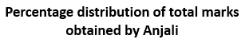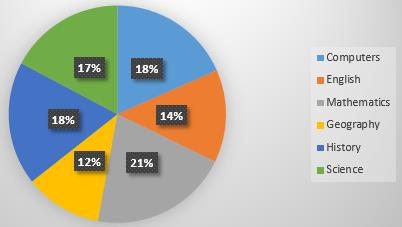Q. How many marks were deducted in the Computers examination?

Solution:

Total marks secured = 500
Marks secured in Computers = 16% of 500 = 80
∵ Maximum marks of each subject = 100
∴ Marks deducted in Computers = 100 – 80 = 20

QUESTION: 73

Directions: Anjali obtained a total of 500 marks in her examination. The given pie-chart shows the percentage distribution of her marks in various subjects. The maximum marks of each subject are 100. Study the pie-chart and answer the questions that follow.Q. In order to get 75% marks in English, how many more marks did she require?

Solution:

Total marks secured = 500
Marks secured in English = 12% of 500 = 60
∵ Maximum marks of each subject = 100
Marks required to get 75% = 75% of 100 = 75
∴ More marks required = 75 – 60 = 15

QUESTION: 74

Directions: Anjali obtained a total of 500 marks in her examination. The given pie-chart shows the percentage distribution of her marks in various subjects. The maximum marks of each subject are 100. Study the pie-chart and answer the questions that follow.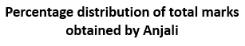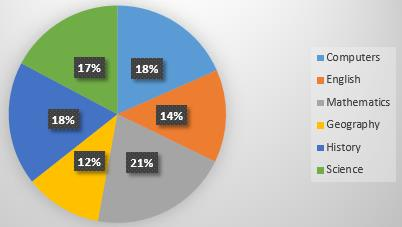Q. How many more marks did she require to score 80% in her examinations?

Solution:

Total marks secured = 500
∵ Maximum marks of each subject = 100
No. of subjects = 7
⇒ Maximum marks = 7 × 100 = 700
⇒ Marks required to score 80% = 80% of 700 = 560
∴ Marks required = 560 – 500 = 60

QUESTION: 75

Directions: Anjali obtained a total of 500 marks in her examination. The given pie-chart shows the percentage distribution of her marks in various subjects. The maximum marks of each subject are 100. Study the pie-chart and answer the questions that follow.Q. What is the difference between the marks obtained in History and Geography?

Solution:

Total marks secured = 500
Marks secured in History = 16% of 500 = 80
Marks secured in Geography = 10% of 500 = 50
∴ Required difference = 80 – 50 = 30

QUESTION: 76

Who is likely to be India’s first anti-corruption ombudsman or Lokpal?

Solution:
• Pinaki Chandra Ghose is likely to be India’s first anti-corruption ombudsman or Lokpal.
• He is the former Supreme Court judge and current member of the National Human Rights Commission (NHRC).
• The Lokpal Act passed in 2013 provides for setting up of Lokpal at the center and Lokayuktas in the States to probe corruption complaints against top functionaries and public servants.
QUESTION: 77

Recently, Manohar Parrikar passed away. He served 2 times as Chief Minister of ________.

Solution:
• Goa Chief Minister Manohar Parrikar passed away in Panaji on 17 March 2019.
• He was diagnosed with pancreatic cancer in February 2018.
• He served as Chief Minister of the Goa from October 2000 to February 2005, and March 2012 to November 2014.
• From November 2014 till March 2017, he served as the Union Defence Minister.
• He served as a Rajya Sabha MP from November 2014 to September 2017.
QUESTION: 78

Indian roller skaters bagged ________ medals in Special Olympics 2019.

Solution:
• Special Olympics World Games 2019 is being held from March 14-21, 2019 in UAE.
• It made history by welcoming a record-breaking 200 nations ahead of the Games.
• Of the 200 nations, 195 will compete in the Games and 5 will observe.
• It is being held in West Asia for the first time. 7,500 athletes will take part in 24 Olympic-style sports for over 7 days.
• Indian roller skaters bagged 49 medals in Special Olympic 2019. India got the maximum number of gold medals in roller skating with 13 gold, 20 silver, and 16 bronze medals.
• Venue: Zayed Sports City Stadium, Abu Dhabi, United Arab Emirates.
QUESTION: 79

Who has been appointed as the Cultural and Tourism Ambassador of Uzbekistan in India in March 2019?

Solution:
• Popular Indian fashion designer Ritu Beri has been appointed as the Cultural and Tourism Ambassador of Uzbekistan in India by the Ministry of Tourism of Uzbekistan.
• This is in recognition of her contribution towards bringing the two countries together by way of fusing cultures in her clothing line.
• She is the first Indian to be appointed to this position.
QUESTION: 80

In human body an element is not found, but is free in nature

Solution: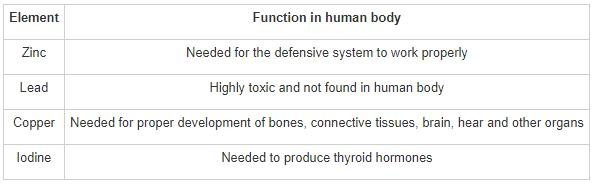QUESTION: 81

AB blood group can take blood from _______.

Solution: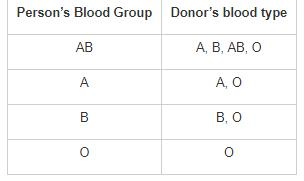QUESTION: 82

Who invented vaccination for small pox?

Solution: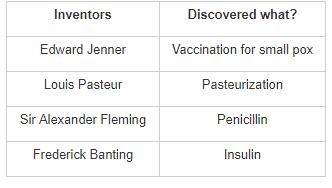QUESTION: 83

A body is said to be under balanced forces when the resultant force acting on the body is _______.

Solution:

Forces are said to be balanced when two equal and opposite forces act on an object which makes net force to be zero. When the Balanced forces are acting the object will be at rest. If the object starts moving then it means that the forces are unbalanced and the object starts moving in the direction of the higher force.

QUESTION: 84

Match the following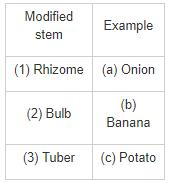Solution:

Rhizome-Banana: The rhizome is the banana plant's stem.

Bulb-Onion: Onion is also known as the bulb onion or common onion. It is a vegetable that is the most widely cultivated species of the genus Allium.

Tuber- Potato: A tuber is the thickened part of an underground stem of a plant potato.

QUESTION: 85

Chinnaswamy cricket stadium is situated in

Solution:

M. Chinnaswamy cricket stadium is located in Bengaluru, Karnataka. It is one of the most prominent cricket stadiums in India along with Eden Gardens (Kolkata), Wankhede (Mumbai).

QUESTION: 86

The size of the image formed by a plane mirror is always _____ to/than the object.

Solution:

The magnification of a plane mirror is always 1 and it forms images which are always of the same size as the object. This because the linear magnification produced by a mirror is equal to the ratio of the image distance to the object distance, with a minus sign.

QUESTION: 87

Which of these Bollywood actresses is the author of the book 'The Diary of a Domestic Diva'?

Solution:

Shilpa Shetty is the author of the book 'The Diary of a Domestic Diva'. The book was published in January 2018.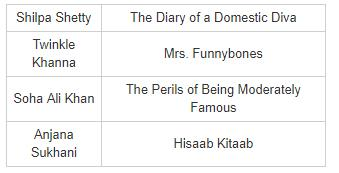QUESTION: 88

‘Knot’ is used to measure.

Solution: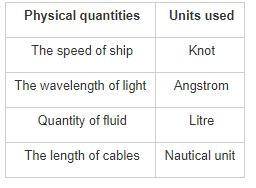QUESTION: 89

Who was the first Indian to reach Antarctica?

Solution:

Antarctica lies in the Southern Hemisphere. Lt. Ram Charan was the first Indian to reach Antarctica under the guidance of Dr Syed Zahur Qasim, Secretary of Department of Environment and former Director of NIO, in 1982.

QUESTION: 90

Who of the following was the architect of Hawa Mahal?

Solution:
• Lal Chand Ustad was the architect of Hawa Mahal.
• Hawa Mahal is a palace located in Jaipur and was built in 1799 by Maharaja Sawai Pratap Singh.
• Hawa Mahal is a palace in Jaipur, India. It is constructed of red and pink sandstone.
QUESTION: 91

In which year was ‘Adhai Din Ka Jhonpda’ built?

Solution:
• Adhai Din Ka Jhonpra is a mosque in the Ajmer. It was commissioned by Qutb-ud-Din-Aibak, on orders of Muhammad Ghori, in 1192 CE.
• It was completed in 1199 CE, and further beautified by Iltutmish in 1213 CE.
QUESTION: 92

Which of the following terms is used in India for a tract of land lying between two confluent rivers?

Solution:

A Doab is a term used in India and Pakistan for the water-rich tract of land lying between two converging, or confluent, rivers. It is similar to an interfluve.

QUESTION: 93

Fossil fuels are most abundantly found in which region of the world?

Solution:
• The Middle East has the most reserves of fossil fuels with about 260 billion reserves in Saudi Arabia, 136 billion reserves in Iran, and 120 billion reserves in Iraq and Kuwait.
• Fossil Fuels are formed by way of natural processes such as decomposition of dead organisms over a long period of time.
• Petroleum, natural gas etc. are the most common examples of fossil fuels.
QUESTION: 94

The coasts of Tamil Nadu and Andhra Pradesh are known by the name of ________.

Solution: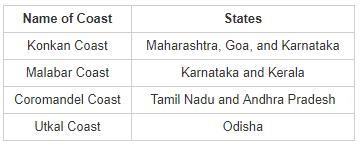QUESTION: 95

'Smelite' is an ore of which of the following metal?

Solution:
• Smelite is an ore of Cobalt (Co) with chemical formula CoAsS2.
• Ore is a naturally occurring rock or mineral from which metals can be extracted.
QUESTION: 96

Tanami  Desert is situated in which among the following continents of the world?

Solution: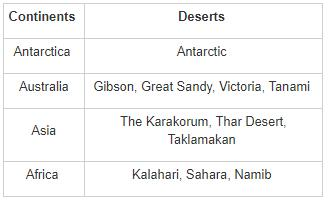QUESTION: 97

Which among the following is a crop that is not grown on laterite soil in India?

Solution:
• Laterite soil is found along the edge of the plateau in the east covering small parts of Tamil Nadu, Odisha and a small part of Chotanagpur in the north and Meghalaya in the north-east.
• It is unsuitable for agriculture but crops such as coffee, rubber, cinchona, cashew, coconut, etc. are grown on this soil.
• This soil is formed by weathering of laterite rocks in high temperatures and heavy rainfall with alternate dry and wet spells.
QUESTION: 98

Who among the following is/are NOT a part of the electoral college for election of the Vice President?

Solution:
• State Legislatures do not take part in the election of the Vice President.
• The electoral college for Vice President consists of the members of the both Houses of the Parliament, according to Article 66 of the Indian Constitution.
• The Vice President is elected by means of a single transferrable vote.
• No formal impeachment is required to be carried out to remove the Vice President from his post.
QUESTION: 99

Which among the following is correct regarding the remuneration of the Advocate General of a state?

Solution:
• The Advocate-General is the highest ranking law officer of a state.
• He is the person who represents the state government in various courts of law.
• Though he is not a member of the state legislature, he can speak in both the houses of the legislature as per the provisions of Article 177 of the Indian Constitution.
• He is appointed for the duration as per the pleasure of the Governor and he also receives such salary as decided by the Governor of the state.
QUESTION: 100

Given below are the facts about the Indian Constitution. Identify the INCORRECT fact.

Solution:
• The Constituent Assembly adopted the national flag on 22 July 1947.
• The Constituent Assembly adopted the national anthem on 24 January 1950. Also, the national song was adopted on the same day.
• Part 20 (Article 368) of the Indian Constitution describes the procedure of Amendment of the Constitution.
• Prem Behari Narain Raizada wrote the original Indian constitution in a flowing italic style with beautiful calligraphy. Artists from Shantiniketan carved and decorated each page.